Probability based design of concrete mixes with cow-bone ash admixed cement

Mahmud ABUBAKAR*, Mohammed ABDULLAHI, and James Isiwu AGUWA

Civil Engineering Department, Federal University of Technology, Gidan Kwano Campus,

P. M. B 65, Minna Niger State Nigeria

E-mail: mahmud1879@futminna.edu.ng (* Corresponding author)

* Corresponding author; Phone +2348037897726

Abstract

A probability-based procedure for design of concrete mixes with cow-cone ash admixed cement has been developed considering the strength as a random variable. However, the compressive strength of concrete in turn, depends on the properties of its constituent materials: cement, fine aggregate, coarse aggregate and cow-bone ash. The compressive strength data generated experimentally has been analysed using normal-probability distribution functions based on 95% confidence interval. The proposed probability based design was compared to the method of trial mixture. It was observed that for reliability index (β) of 1.3, the proposed probability methodology uses 10.2% less cement content than the method of trial mixture; thus, indicating that the method is conservative. It was also observed that, for concrete grade 25, the proposed methodology gives higher compressive strength at both 7 and 28 days. Hence, the probability-based design procedure was found appropriate and is therefore recommended for the design of concrete mixes with cow-bone ash admixed cement.

Keywords

Probability; Green Environment; Concrete Mixes; Cow-Bone Ash

Introduction

The high cost of cement, used as binder, in the production of concrete and the need for a green environment has led to a search for alternatives which have so far centred on the partial replacement of cement with different materials, mostly, agricultural and industrial by-products. In recent times, partial replacement of cement with cow-bone ash is well documented and recommended.

Cow-Bone cause serious disposal problem and continue to accumulate at increasing rates, which if not properly managed, the bone will create increasing environmental problems . For that reason, utilization of the bone as sustainable material in concrete production would help to preserve natural resources and maintain ecological balance. Many studies have been conducted to determine the mechanical properties of both fresh and hardened concrete partially replaced with cow-bone ash [2-7]. Most of the studies revealed that the compressive strength increases up to 10% cow bone ash replacement and from then, decreases as the percentage cow bone ash increases.

Limited studies have been published on the structural safety and or adequacy of using cow bone ash as replacement for cement in concrete production, hence the need to assess the performance of this concrete with the aim of establishing confidence in its utilisation in concrete production and construction industry at large.

Material and method

The materials used in the mixes were ordinary Portland cement , river sand, mineral coarse aggregate, cow bone ash and water. The specific gravities of coarse, fine and bone ash are 2.65, 2.68 and 2.81 respectively while the fineness modulus coarse and fine aggregates are 3.6% and 4.3% respectively.

Cow-Bone was obtained from the abattoir in Minna. The Cow bones were sun-dried after careful separation from flesh, tissues, and fats. The ash was carried out by incinerating the bone at a temperature of 900ºC in a furnace . Only the organic matter was destroyed leaving a white mass of bone salt. The white mass of bone salt was reduced to a fine powder using mortar and piston. The reduced fine powder was further passed through 75µm size sieve to obtain finer particles; the ash was whitish in colour. The fraction passing through 75μm was packed in polythene bags to prevent water absorption and for water proofing and stored in a cool dry place.

The experimental plan has been designed to generate compressive strength data for probabilistic analysis and subsequent development of probability based design procedure. The concrete mixes have been proportioned for different values of parameters which affect the compressive strength of concrete. The range of values of the parameters involved is given in Table 1.

Table 1. Range of values of various parameters

 Parameters Range Water-Cement ratio (x1) 0.55-0.65 Coarse aggregate-Total aggregate ratio (x2) 0.50-0.70 Total aggregate-Cement ratio (x3) 6.0-9.0 Bone Ash-Cement ratio (x4) 0.10-0.40 Workability Medium

The water content requirement for aggregates and other values has being selected based on the workability and durability requirements.

Concrete mix proportions

MINITAB14 (2004) was used to determine 31 different mix proportions. Various adjustments due to variations in the specific gravity and water absorption were also incorporated in the design. The mixes were designed for medium workability. Central composite design was employed to limit the number of experimental runs compared to factorial design. This enables modelling of mixture proportions involving interaction and quadratic terms. These models were used for optimisation of concrete mixes for given criteria of interest.

The factors used in this work are as given in Table 1. The Minitab software was used for the factor setting as shown in Table 2.

Table 2. Factor Setting using Central Composite Design

 Factors Axial Point Minimum Centre Maximum Axial Point Coded Values X1 -1.414 -1 0 +1 +1.414 X2 -1.414 -1 0 +1 +1.414 X3 -1.414 -1 0 +1 +1.414 X4 -1.414 -1 0 +1 +1.414

Factors defined in Table 1.

The coded values from the software are converted to uncoded or actual values using equation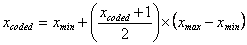(1)

where xcoded is the uncoded values, xmin is the uncoded minimum values (corresponding to -1), xminis the uncoded maximum values (corresponding to +1), and xcoded is the coded value to be translated to uncoded value. The uncoded values were converted to batch quantities in kg/m3. The mix proportioning was done using absolute volume method.

Casting of concrete cubes

Five (5) cubes of 150×150×150mm size were cast for each of the 31 mix designs, these cubes were tested at 28 days of curing period. For all these mix proportions, required quantities of materials were weighed.

Statistical parameters

The results of the compressive strengths, for each mix, are presented in the form of mean, standard deviation and within-test coefficient of variation. The within-test coefficient of variations is calculated using Equation 1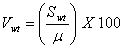(2)

where Vwt is within-test coefficient of variation expressed as per cent, µ is the average strength in N/mm2 and Swt is the within-test standard deviation given by Equation 2;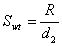(3)

where R is the average range for all tests of a class of concrete and is the difference between the highest and lowest strengths of the cubes making up a test, and d2 is a factor based on number of cubes within the test. The values of d2 are given in ACI 301 .

Mathematical model

Reliability checks on structural elements involve the evaluation of reliability index, β, or evaluating the safety equivalent of a particular reliability index. The probability-based design procedure is a reverse case, wherein a design has to be achieved which shall ensure a certain level of reliability. In this work, a design procedure has been developed using the first order second moment approach using Hasofer-Linds method. The procedure involves the calculation of design parameters for a given reliability index, β. Different levels of reliability give different surfaces, resulting in different designs. Hence, in the proposed probability-based design, the design values of the variables, which will ensure that the design have a failure surface that complies with a required reliability index, β, are determined.

Using the with-in-test coefficient of variation for compressive strength, the revised coefficients of variation and standard deviation have been calculated using the equation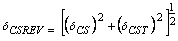(4)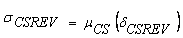(5)

where: δCSREV = revised values of coefficient of variation; σCSREV = revised values of standard deviation; δCS = experimentally obtained values of coefficient of variation; δCST = within test coefficient of variation; μCS = means of compressive strength

Taking the coefficient of variation of w/c, and b/c ratios at site to be 5%, thus, the standard deviation values for water-cement ratio wcr) have been obtained by multiplying the actual values by the coefficient of variation. The values of the means, revised coefficients of variation and standard deviations of compressive strengths for various water cement, coarse aggregate cement, total aggregate cement, and bone-ash cement ratios have been calculated and presented.

Normal distribution function

The design values are governed by the equation below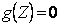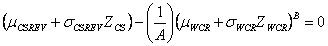(6)

The values of α1, α2, and α3 have being evaluated using the equations presented bellow: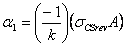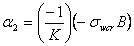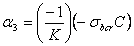(7)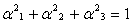(8)

Using these values of α1 α2 and α3, the design compressive strength and water cement ratio for different reliability indices (β= 1.0, 1.3, 2.0, and 3.0) are obtained from the equations below: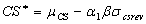(9)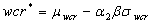(10)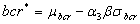(11)

The relationship between compressive strength and water-cement ratio, and water-cement ratio and aggregates proportion are represented in the form of design curves.

Systematic procedure for proportioning concrete mix

(i) Characteristics of aggregates

The first step is to determine the properties of available coarse and fine aggregates.

(ii) Determination of target mean compressive strength of concrete

This depends upon the confidence level or the reliability index, and the degree of quality control expected at site. The reliability index is chosen based on the importance of structure.

Thus, the target strength is: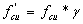(12)

where f’cu= the target mean strength; fcu= the desired 28-day strength at site γ = modification factor.

(iii) Selection of water-cement ratio

For the desired target mean strength, and the reliability index, the water-cement ratio is selected.

(iv) Determination of water content

Based on the workability requirement for a particular job, the water content is selected

(v) Determination of fine aggregate-cement ratio

The total aggregate-cement ratio corresponding to the water-cement ratio selected above (iii) is determined from reference curve. Water cement ratio is then compared with the maximum free water cement ratio allowed from durability considerations. The minimum of the two values is chosen for further design calculations.

(vi) Determination of coarse aggregate to cement ratio

For the revised water-cement ratio obtained in (iv), the coarse aggregate to cement ratio, aca is determined. The aggregates to cement ratios are adjusted as explained below for any difference in specific gravities of fine and coarse aggregates from the reference values .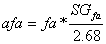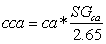(13)

where (SG)fa and (SG)ca are the specific gravity values of the available fine and coarse aggregates respectively.

(vii) Calculation of cement content

The cement content is obtained from the water content obtained in step-iv.

(viii) Calculation of quantities of materials

The contents of cement material, water, fine and coarse aggregates per unit volume of concrete were estimated based on the values obtained in the earlier steps.

Results and discussion

Proposed design curves

It is observed that the curves derived from the probability or safety checks shown in Figures 1 to 4 give strengths with equal proportion and with small variation between each other because of the small range of values considered for the water-cement ratio. It is also observed that the higher the reliability index, the lower the water-cement ratios to take care of the reduction in probability of failure.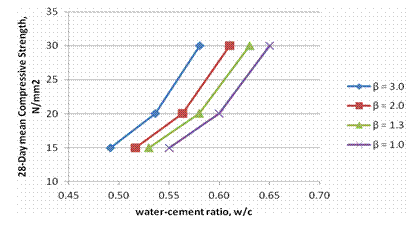Figure 1. Design curves for 28-day compressive strength for various reliability indices (β) (Source of data: Field work, 2015)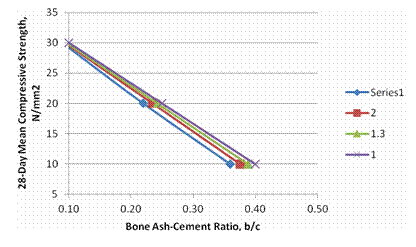Figure 2. Design curves for 28-day compressive strength for various reliability indices (β) (Source: Field work, 2015)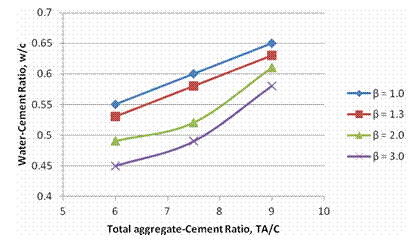Figure 3. Variation of total aggregates-cement ratio with water-cement ratio for various reliability indices (β) (Source: Field work, 2015)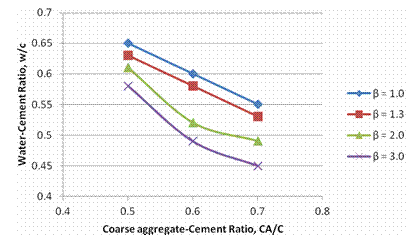Figure 4. Variation of coarse aggregate-cement ratio with water-cement ratio for various reliability indices (Source: Field work, 2015)

Concrete mix design example

Design parameters and the comparisons of resulted materials are presented in Table 3, respectively Table 4.

Table 3. Design parameters

 Characteristic compressive cube strength at 28-days 25N/mm2 Reliability Index (multi-Storey residential building) 1.3 Maximum size of aggregate 20mm Degree of workability Medium Degree of quality control Very good Type of exposure Mild

Table 4. Comparison of material quantity in kilogram

 Mix design procedure Water (kg) Cement (kg) FA (kg) CA (kg) BA (kg) Proposed probability based design procedure 168.0 271.0 1095.4 1235.2 54.0 ACI Method 168.0 298.6 842.7 1067.0 74.7

FA: Fine aggregates, CA: Coarse aggregates, BA: Bone ash

Validation

Twenty (20) cubes with the proportion of materials estimated using the proposed design methodology as well as the method of trial mixture were cast. The compressive strengths of the cubes tested at 7 and 28 days curing are given in Figure 5.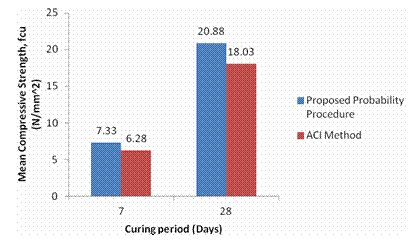Figure 5. Mean compressive strength comparison

Concrete mixes proportioned for higher reliability index require lower water-cement ratio to ensure reduced probability of failure. However, concrete mixes with bone ash cement ratio of 0.1 have more strength than concrete mixes with higher ratios indicating that the higher the cow bone ash content beyond 10%, the lower the strength of the concrete.

Furthermore, the proposed design procedure uses 10.2% lesser cement content when compared with the ACI method. Also, the proposed probability-based mix attains higher strength at both 7 and 28 days, thus, ACI method which uses higher content of cement is highly conservative.

In addition, the physical properties of the aggregates obtained/used are within the range for natural aggregates [12-14].

Conclusions

The following conclusions can be drawn from this study:

(1)     The proposed reliability based design methodology uses 10.2% less cement content than the ACI method indicating that the ACI method of trial mixture is conservative.

(2)     For a particular concrete grade, the proposed methodology gives higher compressive strength at both 7 and 28 days.

(3)     Probability-based design procedure was found appropriate and is therefore recommended for the design of concrete mixes with cow-bone ash admixed cement

References

1.      Chukwu O., Adeoye P. A., Chidiebere I., Abattoir wastes generation, management and the environment: a case of Minna, North Central Nigeria, International Journal of Biosciences (IJB), 2011, 1(6), p. 100-109.

2.      Adabaa C. S., Agunwamba J. C., Nwojic C. U., Onya O. E., Ozeh S., Comparative Cost and Strength Analysis of Cement and Aggregate Replacement Materials, Nigerian Journal of Technology, 2012, 31(2), p. 111-115.

3.      Akhionbare W. N., A Comparative Evaluation of the Application of Agro waste as Construction Material, International Journal of Science and Nature, 2013, 4 (1), p. 141-144.

4.      Bhat J. A., Qasab R. A., Dar A. R., Machine Crushed Animal Bones as Partial Replacement of Coarse Aggregates in Lightweight Concrete, ARPN Journal of Engineering and Applied Sciences, 2012, 7(9), p. 1202-1207.

5.      Falade F., Ikponmwosa E., Fapohunda C., Environmental Benefits of the Structural Use of Pulverized Bone in the Production of Foamed Aerated Conrete Journal of Emerging Trends in Engineering and Applied Sciences, 2013, 4(5), p. 717-722.

6.      Falade F., Ikponmwosa E., Fapohunda C., Potential of Pulverized Bone as a Pozzolanic material, International Journal of Scientific & Engineering Research, 2012, 3(7), p. 1-6.

7.      Manasseh J. A., Review of Partial Replacement of Cement with Some Agro Wastes, Nigerian Journal of Technology, 2010, 29(2), p. 12-20.

8.      BS 12, Specification for Portland cement. British Standard Institution, Her Majesty Stationary office 1996, London.

9.      ACI 214R, Guide to Evaluation of Strength Test Results of Concrete, American Concrete Institute, 2002.

10.  ACI 301, Specification for Structural Concrete with Selected ACI References, American Concrete Institute, Farmington Hills, 2005.

11.  Kumar M., Reliability based design of structural elements, Ph.D. Thesis, Civil Engineering Department, Thapar Institute of Engineering and Technology, Patiala, Punjab India, 2002.

12.  Abdullahi M., Properties of Some Natural Fine Aggregates in Minna, Nigeria and Environs, Leonardo Journal of Sciences, 2006, (8), p. 1-6.

13.  ASTM C127, Test Method for Density, Relative Density and absorption of Coarse Aggregate, Annual Book of ASTM Standards, 2012.

14.  ASTM C136, Test Method for Sieve Analysis of Coarse and Fine Aggregates, Annual Book of ASTM Standards, 2006.